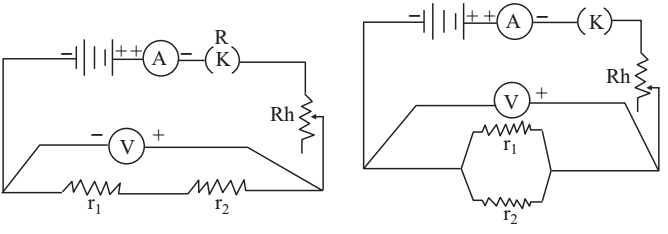# Law of Combination of Resistances Experiment

Experiment: To verify the law of combination (series and parallel) of resistances using ammeter - voltmeter method and coils of known resistances.

According to Ohm’s law, when a steady current I flows through a conductor the potential difference across its ends is directly proportional to it, provided that the physical conditions remain the same.

V ∝ I

V = RI

When two or more resistances are connected in series then the net resistance of such a combination is equal to the sum of the individual resistances. If two resistances r1 and r2, are connected in series, then net resistance Rs is given by the relation

Rs = r1 + r2

When two or more resistances are connected in parallel the reciprocal of the total resistance of such combination is equal to the sum of the reciprocals of the individual resistances. For two resistances r1 and r2 connected in parallel, the net resistance RP of the combination is given by

1/RP = 1/r1 + 1/r2

Material Required

A battery, an ammeter, a voltmeter, rheostat, one way key, sand paper, coils of known resistance and connecting wires

### How To Perform Experiment

1. Determine the least count of the voltmeter and ammeter, and note the zero, error, if any.

2. Draw the electrical circuit in your copy and make connections according to it. Make sure that connections are neat and tight and insulation from ends of the wires is properly removed by sand paper.

3. Insert the key K, slide the rheostat contact and thus see that ammeter and voltmeter are working properly.

4. Observe the zero error of each instrument by making the current-zero by taking out the key K. Then again insert the key K.

5. Adjust the sliding contact of the rheostat such that either ammeter or voltmeter gives full scale deflection reading. Deflection in the other instrument also should be more than half of the full scale deflection.

6. Note down the value of potential difference V from voltmeter and current I from ammeter.

7. Take at least three sets of independent observations.

8. Repeat the whole experiment for parallel arrangement.### Sources of Error

1. The connections may be loose.
2. There may be some insulation at the ends of connecting wires left over even after cleaning them by sand paper.